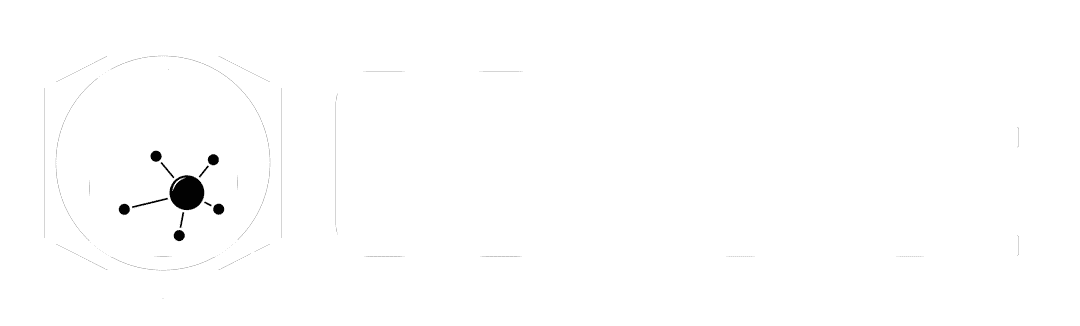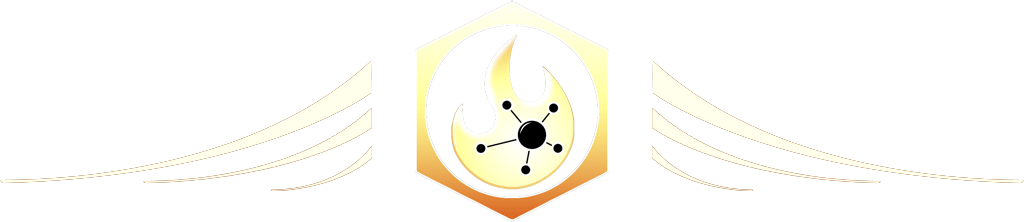# Attribute Randomize

The Ultimate Houdini node reference

# Visit the Node Bible

### Attribute Randomize

#### The Attribute Randomize node modifies/creates attributes based on random values of various distributions.

Drag to resize
Summary:

The attribute randomize node is great for initializing or modifying existing attributes by random values. They key to understanding this node is understanding what the various distributions do. These distributions are basically different algorithms which create random values.
One really cool way of seeing the resulting random values is by toggling the "Visualize as Points" parameter in the options tab. What this will do is plot a point's position to the randomized distribution.
As an example, if you want to see what the "Inside Sphere" distribution does, then select that under distribution and check on "Visualize as Points." By default, it will create 10,000 points that exist in a spherical shape between -1 and 1 on x,y, and z. The random positions of those points will represent the noise values generated by that distribution.

Drag to resize

Main Parameters:

Group:

--  This allows you to only apply that attribute to certain points, primitives, or vertices of your mesh.

Attribute Class:

--  What sort of attribute would you like to create?  Point? Vertex? Primitive?  If these terms are unfamiliar to you, then please visit Houdini For the New Artist.

Attribute Name:

--  What would you like to name the new attribute?  Alternatively, if you are modifying an existing attribute, then put its name here.

Operation:

--  If an existing attribute exists, then how would you like to blend the old value with the values generated by the distribution?  You can add, multiply, go with the minimum value between the old/new, or go with the max value between the old/new attributes.

Global Scale:

--  Increases both the min/max values which the distribution is allowed to generate.  In other words, if a distribution is allowed to create values between -1 and 1, then scaling up might change that min/max to -3 and -3.  If the distribution values are between 0 and 1, then scaling up would allow values to go from 0 to 3.  Again, to see this properly, use the "Visualize as Points" setting.

Drag to resize

Distributions:

Uniform (Continuous):

--  Random values are generated in-between a min and a max range with equal probability for all values in that range.  Includes decimal values.
Constant Value (not random):

--  Generates a non-random value.  Not quite sure why this exists in the "randomize attribute" node, but it does.

Two Values:

--  Generates a random probability that the value will be either Value A or Value B.  You can also determine the probability of both by adjusting the probability of Value B (the probability of A will obviously be the remaining percentage to reach 100%).

Uniform (discrete):

--  Random values are generated between the min and max.  In addition, the values need to be a multiple of the step size.  It cannot exist as just any float value between the min and max.  It can only increase or decrease in value by the step size

Direction or Orientation:

--  Random values are generated one unit away from 0 in random directions. (with the exception of setting the dimension to 4)  Take a look at the video below for the best understanding of what this distribution can do.

Inside Sphere:

--  See above for the "Direction or Orientation."  The only difference between that distribution and "Inside Sphere" is the fact that values will not always be one unit away from 0.  In addition, if you set the dimensions to 4, the quaternion behavior is slightly different.

Normal (Gaussian):

-- When choosing, Normal (Gaussian), Exponential, Log-Normal, or Cauchy-Lorentz options, it's best to preview these values first in 2 dimensions before bringing it to 3.  Check out the videos below for an overview of all these various options.  They're best understood visually.

Custom Discrete:

--  This setting allows you to specify specific values along with their relative weight/probability of distribution.

As an example, lets say that you want to spawn various trees and control the likelihood of them spawning depending on the species.  Each species could have an id that corresponds with a value that you set in the attribute randomize node.  And, that the likelihood of that value being chosen can be controlled by the weight.

Drag to resize

Misc Options

Visualize as Points:

--  This setting is designed to help you understand the resulting random values visually.  The way it does so is by creating a certain number of points and plotting their position based on the output of the random distribution algorithm that you've selected.

Fraction Attribute:

--  In order for this to work, you need to feed the Attribute Randomize node a float attribute called "fraction."  This fraction will essentially cause certain values to only appear along certain areas of of the distribution.  The user docs contains a useful mathematical definition of this as well:

"This gives a way of non-randomly sampling the distribution, by specifying locations in the uniform parametric space of the distribution, known as "quantiles".
For example, if the fraction attribute is 0.61 for some element, and the distribution is continuous and 1-dimensional, the resulting value should be greater than exactly 61% of samples, if one were to sample the distribution an infinite number of times. For multi-dimensional distributions, the fraction attribute should have as many components as are needed to represent the distribution. For example, when sampling directions in 3D, it should have size 2, corresponding (non-linearly) with longitude and latitude."

See the video below for a demonstration.
Global Seed:

--  Gives you a different version of the distribution values.  This is not applicable when using the Fraction Attribute because, in that situation, the fraction attribute gets used as the seed.

Seed Attribute:

--  This parameter will ensure that the seed does not change along with changing point numbers.  This is especially important when dealing with particle simulations which feature particles that die.  When particles die, their point IDs change, and this could cause the attribute randomize to assign different values when those ids change.  Enabling a seed attribute will prevent this from occurring because it associates the version of distribution values with an id attribute instead of point numbers.  This is not applicable when using the Fraction Attribute because, in that situation, the fraction attribute gets used as the seed.